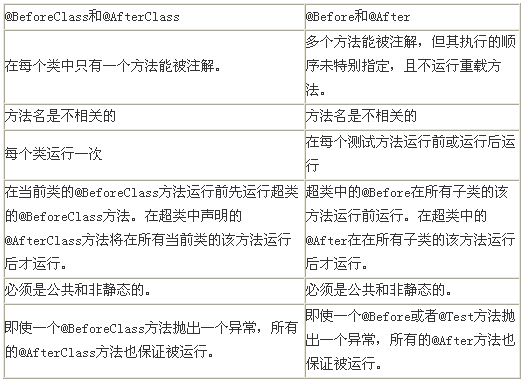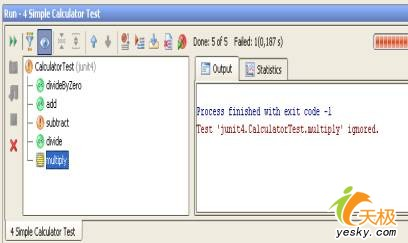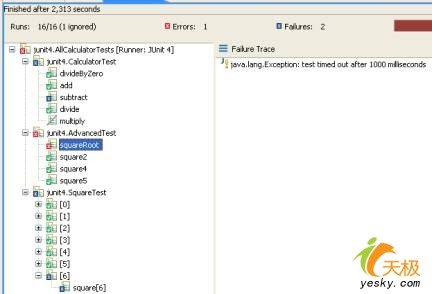UML软件工程组织

 全面认识JUnit 4的新特征 作者：朱先忠编译 出处：天极开发

JUnit 4是一种与其之前的版本完全不同的API，它根据Java 5.0中的新特征(注解，静态导入等)构建而成。如你所见，JUnit 4更简单、更丰富和更易于使用，而且它引入了更为灵活的初始化和清理工作，还有限时的和参数化测试用例。

 package calc; public class Calculator { 　private static int result; //存储结果的静态变量 　public void add(int n) { 　　result = result + n; 　} 　public void substract(int n) { 　　result = result - 1; //错误：应该是"result = result - n" 　} 　public void multiply(int n) {} //还没实现 　public void divide(int n) { 　　result = result / n; 　} 　public void square(int n) { 　　result = n * n; 　} 　public void squareRoot(int n) { 　　for (; ;) ; //错误：无限循环 　} 　public void clear() { //清除结果 　　result = 0; 　} 　public void switchOn() { //打开屏幕，显示"hello"，并报警 　　result = 0; //实现其它的计算器功能 　} 　public void switchOff() { } //显示"bye bye"，报警，并关闭屏幕 　public int getResult() { 　　return result; 　} }

JUnit 3.8

 package junit3; import calc.Calculator; import junit.framework.TestCase; public class CalculatorTest extends TestCase { 　private static Calculator calculator = new Calculator(); 　@Override protected void setUp() { calculator.clear(); } 　public void testAdd() { 　　calculator.add(1); 　　calculator.add(1); 　　assertEquals(calculator.getResult()， 2); 　} 　public void testSubtract() { 　　calculator.add(10); 　　calculator.subtract(2); 　　assertEquals(calculator.getResult()， 8); 　} 　public void testDivide() { 　　calculator.add(8); 　　calculator.divide(2); 　　assert calculator.getResult() == 5; 　} 　public void testDivideByZero() { 　　try { 　　　calculator.divide(0); 　　　fail(); 　　} 　　catch (ArithmeticException e) { } 　} 　public void notReadyYetTestMultiply() { 　　calculator.add(10); 　　calculator.multiply(10); 　　assertEquals(calculator.getResult()， 100); 　} }

JUnit 4

 package JUnit 4; import calc.Calculator; import org.junit.Before; import org.junit.Ignore; import org.junit.Test; import static org.junit.Assert.*; public class CalculatorTest { 　private static Calculator calculator = new Calculator(); 　@Before public void clearCalculator() { 　　calculator.clear(); 　} 　@Test public void add() { 　　calculator.add(1); 　　calculator.add(1); 　　assertEquals(calculator.getResult()， 2); 　} 　@Test public void subtract() { 　　calculator.add(10); 　　calculator.subtract(2); 　　assertEquals(calculator.getResult()， 8); 　} 　@Test public void divide() { 　　calculator.add(8); 　　calculator.divide(2); 　　assert calculator.getResult() == 5; 　} 　@Test(expected = ArithmeticException.class) 　public void divideByZero() { 　　calculator.divide(0); 　} 　@Ignore("not ready yet") 　@Test 　public void multiply() { 　　calculator.add(10); 　　calculator.multiply(10); 　　assertEquals(calculator.getResult()， 100); 　} }

三、 包

 java.lang.Exception: No runnable methods.

 public static void assertEquals(String message， Object[] expecteds， Object[] actuals); public static void assertEquals(Object[] expecteds， Object[] actuals);

Fixture是在测试期间初始化和释放任何普通对象的方法。在JUnit 3.8中，你要使用setUp()来实现运行每一个测试前的初始化工作，然后使用tearDown()来进行每个测试后的清理。这两个方法在TestCase类中都得到重载，因此都被唯一定义。注意，我在这个Setup方法使用的是Java5.0内置的@Override注解-这个注解指示该方法声明要重载在超类中的方法声明。在JUnit 4中，则代之使用的是@Before和@After注解；而且，可以以任何命名(在我们的例子中是clearCalculator())来调用这些方法。在本文后面，我将更多地解释这些注解。

JUnit 3.8通过分析它的签名来识别一个测试方法：方法名必须以"test"为前缀，它必须返回void，而且它必须没有任何参数(举例来说，publicvoidtestDivide())。一个不遵循这个命名约定的测试方法将被框架简单地忽略，而且不抛出任何异常（指示发生了一个错误）。
JUnit 4不使用与JUnit 3.8相同的约定。一个测试方法不必以'test'为前缀，但是要使用@Test注解。但是，正如在前一个框架中一样，一个测试方法也必须返回void并且是无参数的。在JUnit 4中，可以在运行时刻控制这个要求，并且不符合要求的话会抛出一个异常：

 java.lang.Exception: Method xxx should have no parameters java.lang.Exception: Method xxx should be void

@Test注解支持可选参数。它声明一个测试方法应该抛出一个异常。如果它不抛出或者如果它抛出一个与事先声明的不同的异常，那么该测试失败。在我们的例子中，一个整数被零除应该引发一个ArithmeticException异常。

 java -ea junit.textui.TestRunner junit3.CalculatorTest ..F.E. There was 1 error: 1) testDivide(junit3.CalculatorTest)java.lang.AssertionError at junit3.CalculatorTest.testDivide(CalculatorTest.java:33) There was 1 failure: 1) testSubtract(junit3.CalculatorTest)junit.framework.AssertionFailedError: expected:＜9＞ but was:＜8＞ at junit3.CalculatorTest.testSubtract(CalculatorTest.java:27) FAILURES!!! Tests run: 4， Failures: 1， Errors: 1

TestDivide产生一个错误，因为断言确定了8/2不等于5。TestSubstract产生一个失败，因为10-2应该等于8，但是在这个实现中存在一个错误：它返回9。

 java -ea org.junit.runner.JUnitCore junit3.CalculatorTest JUnit version 4.1 ..E.E. There were 2 failures: 1) testSubtract(junit3.CalculatorTest) junit.framework.AssertionFailedError: expected:＜9＞ but was:＜8＞ at junit.framework.Assert.fail(Assert.java:47) 2) testDivide(junit3.CalculatorTest) java.lang.AssertionError at junit3.CalculatorTest.testDivide(CalculatorTest.java:33) FAILURES!!! Tests run: 4， Failures: 2 *** java -ea org.junit.runner.JUnitCore JUnit 4.CalculatorTest JUnit version 4.1 ...E.EI There were 2 failures: 1) subtract(JUnit 4.CalculatorTest) java.lang.AssertionError: expected:＜9＞ but was:＜8＞ at org.junit.Assert.fail(Assert.java:69) 2) divide(JUnit 4.CalculatorTest) java.lang.AssertionError at JUnit 4.CalculatorTest.divide(CalculatorTest.java:40) FAILURES!!! Tests run: 4， Failures: 2

十、 高级测试

(一) 高级预设环境Start test system //父类的@BeforeClass Switch on calculator //子类的@BeforeClass Initialize test system //第一个测试 Clear calculator Initialize test system //第二个测试 Clear calculator Clean test system Initialize test system //第三个测试 Clear calculator Clean test system Initialize test system //第四个测试 Clear calculator Clean test system Switch off calculator //子类的@AfterClass Stop test system //父类的@AfterClass

(二) 限时测试

 There was 1 failure: 1) squareRoot(JUnit 4.AdvancedTest) java.lang.Exception: test timed out after 1000 milliseconds at org.junit.internal.runners.TestMethodRunner.runWithTimeout(TestMethodRunner.java:68) at org.junit.internal.runners.TestMethodRunner.运行(TestMethodRunner.java:43) FAILURES!!! Tests run: 4， Failures: 1

(三) 参数化测试

 java org.junit.runner.JUnitCore JUnit 4.SquareTest JUnit version 4.1 .......E There was 1 failure: 1) square(JUnit 4.SquareTest) java.lang.AssertionError: expected:＜48＞ but was:＜49＞ at org.junit.Assert.fail(Assert.java:69) FAILURES!!! Tests run: 7， Failures: 1

(四) 测试集

 package JUnit 4; import org.junit.runner.RunWith; import org.junit.runners.Suite; @RunWith(Suite.class) @Suite.SuiteClasses({ CalculatorTest.class， SquareTest.class }) public class AllCalculatorTests {}

 java -ea org.junit.runner.JUnitCore JUnit 4.AllCalculatorTests JUnit version 4.1 ...E.EI.......E There were 3 failures: 1) subtract(JUnit 4.CalculatorTest) java.lang.AssertionError: expected:＜9＞ but was:＜8＞ at org.junit.Assert.fail(Assert.java:69) 2) divide(JUnit 4.CalculatorTest) java.lang.AssertionError at JUnit 4.CalculatorTest.divide(CalculatorTest.java:40) 3) square(JUnit 4.SquareTest) java.lang.AssertionError: expected:＜48＞ but was:＜49＞ at org.junit.Assert.fail(Assert.java:69) FAILURES!!! Tests run: 11， Failures: 3

(五) 测试运行机

 import org.junit.internal.runners.TestClassRunner; import org.junit.runner.RunWith; @RunWith(TestClassRunner.class) public class CalculatorTest { ... }

十一、 工具集成功能

 public static junit.framework.Test suite() { 　return new JUnit 4TestAdapter(CalculatorTest.class); }

(一) Intellij IDEA

IDEA 5并没有集成JUnit 4。没有办法，我们只好等待IDEA 6中实现这一集成了。在这个例子中，我使用了较早的发行版本(Demetra build 5321)；但是，参数化测试用例仍不能工作。图1展示了这个CalculatorTest的执行情况(被忽略的测试以一个不同的图标标志)。(二) Eclipse

(三) Ant集成

junit任务当前仅仅支持JUnit 3.8风格测试；这也就是说，你还必须用一个JUnit 4 TestAdapter来包装你的JUnit 4测试；这样，它们才能在Ant中运行。这个＜junit＞任务与其在JUnit 3.8中用法一样：

 ＜!-- Test --＞ ＜target name="test" depends="compile"＞ ＜junit fork="yes" haltonfailure="yes"＞ ＜test name=" JUnit 4.AllCalculatorTests"/＞ ＜formatter type="plain" usefile="false"/＞ ＜classpath refid="classpath"/＞ ＜/junit＞ ＜/target＞

 版权所有：UML软件工程组织